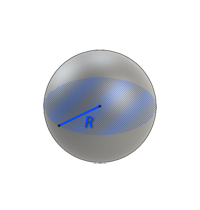# Volume of a sphere

SphereCalculation formula

Formula for calculating the volume of the sphere:

V = 4 · r3 · π / 3,

where r is radius, π = constant equal to (3.14)